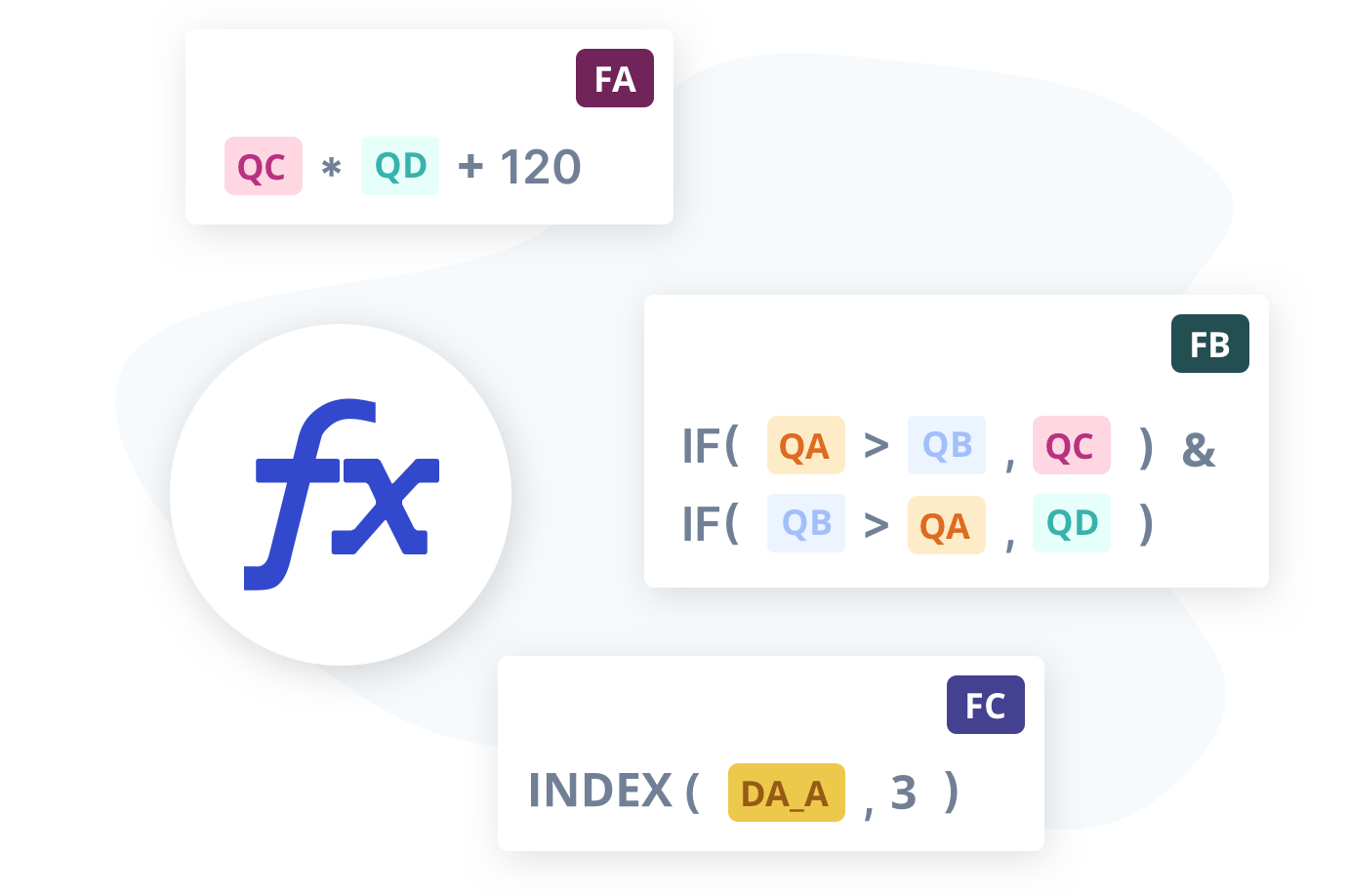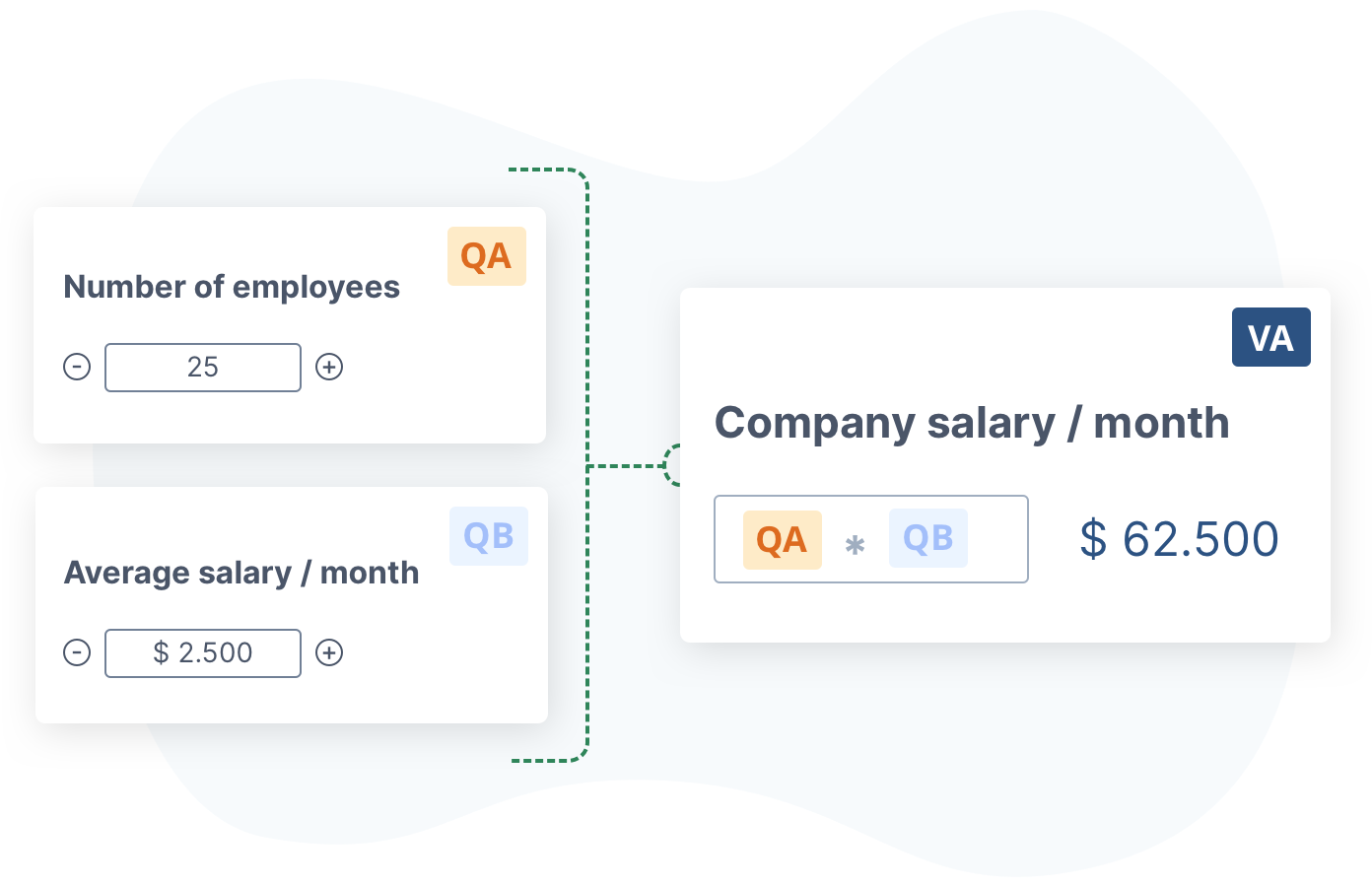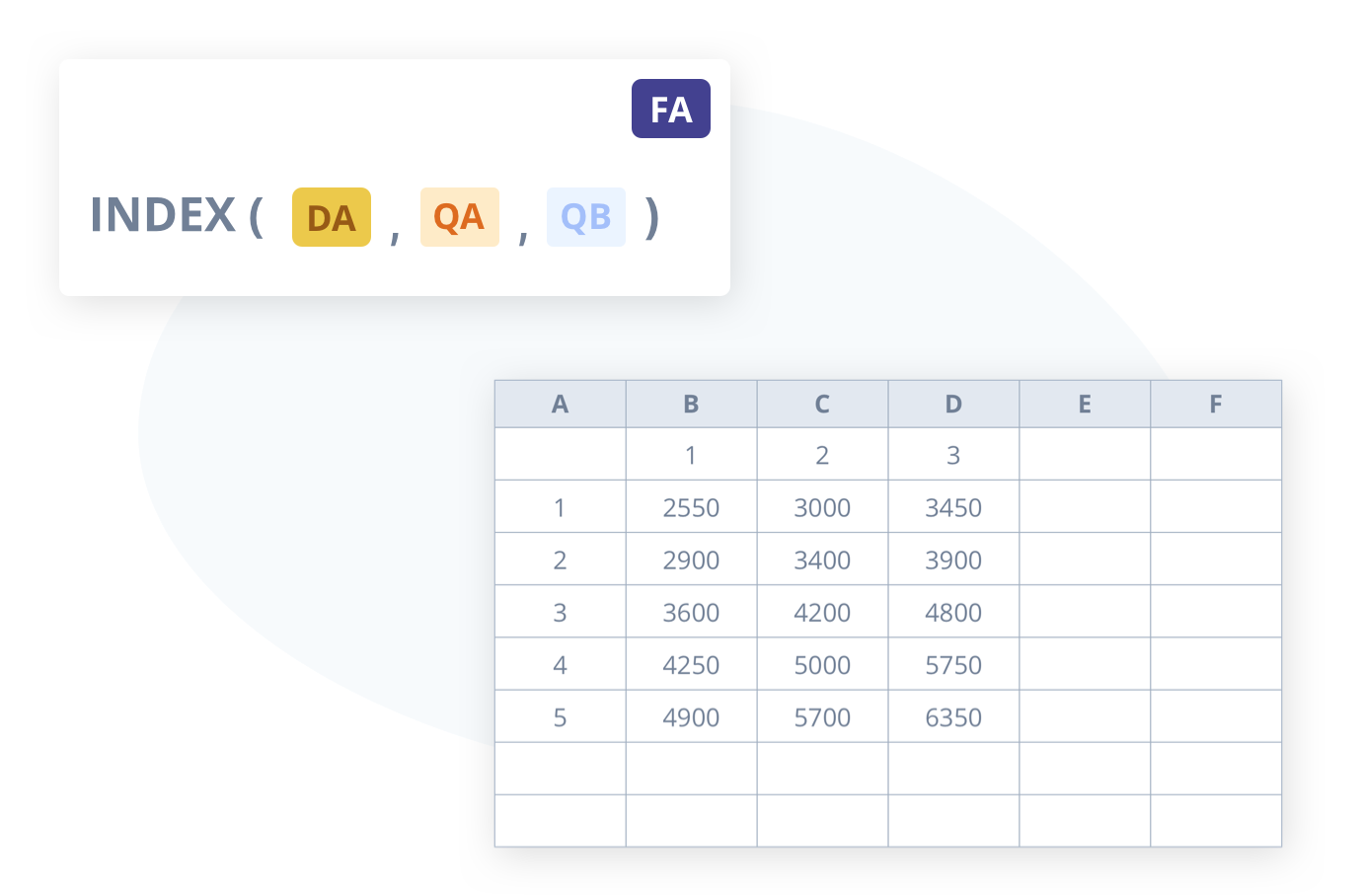## Let your website visitors turn the knobs and calculate their price, ROI or savings in a form on your website

No credit card required
Cancel anytime

4.8 out of 5 stars

4.8 / 5
"Any website needs a calculator!"

ConvertCalculator allows you to create a calculator form for your website to display prices of products, savings, ROI or costs. This enables you to capture more leads quicker and follow-up with confidence. Create advanced formulas in a simple way with our formula builder. Go ahead and experience the sheer power of Excel-type formulas in your web-form. And start capturing more leads!

## Formulas are our power feature

A formula or function is an expression that calculates a value. A function has input arguments, and returns an output. In your calculation-form, the input arguments can relate to the value that is assigned to a form-fields, a variable or a table. Popular functions are the IF, AND and OR functions. With ConvertCalculator nearly anything is possible. So go ahead and put your business logic in your embedded calculation form.## Variables to keep you sane

If your formulas are straightforward, you can get away with re-using bits and pieces in many Formulas. In complex calculation forms, things can get confusing quite fast! For this reason, we have created Variables. Variables are special formulas, that can be re-used in other Formulas (or Variables). Variables keep your Formulas clean. So your head won't explode while building complicated calculation forms.## Need spreadsheets? We've got you covered

Some calculations are tied to a simple input, like the value of a number field. Other formulas need a large dataset as input. That's when it becomes scary. Don't worry though, we've got you covered! Our Datasheets are a similar to Excel spreadsheets. Although simplified by design. You can create a datasheet from scratch. Or copy paste from an Excel file. Once set up, you can use the datasheet in your calculations with functions like INDEX and VLOOKUP.examples

and more

## The Swiss army knife for building calculation forms

### Assign values

Connect your formula to your form inputs by assigning values to elements (e.g. multiple choice, selection or dropdown). Simple, but powerful.

### Formula builder

Create anything you need with our bespoke formula builder. From straightforward to highly complex calculations. We've got you covered.

### External data

Let your website visitors calculate their price, ROI or savings

### What do you mean with 'formula builder'?

The formula builder is our mechanism for creating formulas and variables. You can compare our formula builder to the "formula bar" in Excel. There is one difference though. Cells in Excel can function as an input and an output value. In ConvertCalculator, formulas always display output value. That is why we do not require an '=' symbol before you type. Adding an ' = ' before your formula, will throw an error.

### How do I make a formula with the outputs of two questions?

You can reference the value of a question by including the question reference in the formula. E.g. if you want multiply question A and question B, your formula becomes 'QA * QB'.

For number-questions, the output value is clear. For multiple choice and multiple selection questions, there is value tied to each option. This makes it possible to use these types of questions as well.

### Does ConvertCalculator support functions such as IF?

Yes, ConvertCalculator supports most functions that are used in Excel. This makes it super powerful to use your business logic in a calculator or create decision trees / conditional logic.

For the full list of the functions we support, visit this page